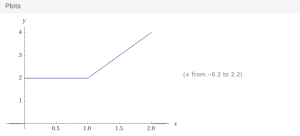Posted on

## Calculus Ch1.1 #44, 46, 48, 50

Here are the solutions to these even-numbered problems.

Calc1-Ch1.1#44-46-48-50.pdf (The PDF has #49 instead of #50. See below for #50)

Chapter 1.1 #50

What you are looking for is a function like
C = f(x) where C is cost and x is mile
If 0 <= x <= 1, C=2
If 1 < x < =2, C = 2 + 0.2(10(x-1))
Using Wolfram here is what it looks like